Related Articles

# Drop rows from Pandas dataframe with missing values or NaN in columns

• Last Updated : 02 Jul, 2020

Pandas provides various data structures and operations for manipulating numerical data and time series. However, there can be cases where some data might be missing. In Pandas missing data is represented by two value:

• None: None is a Python singleton object that is often used for missing data in Python code.
• NaN: NaN (an acronym for Not a Number), is a special floating-point value recognized by all systems that use the standard IEEE floating-point representation

Pandas treat `None` and `NaN` as essentially interchangeable for indicating missing or null values. In order to drop a null values from a dataframe, we used `dropna()` function this function drop Rows/Columns of datasets with Null values in different ways.

Syntax:
DataFrame.dropna(axis=0, how=’any’, thresh=None, subset=None, inplace=False)

Parameters:
axis: axis takes int or string value for rows/columns. Input can be 0 or 1 for Integer and ‘index’ or ‘columns’ for String.
how: how takes string value of two kinds only (‘any’ or ‘all’). ‘any’ drops the row/column if ANY value is Null and ‘all’ drops only if ALL values are null.
thresh: thresh takes integer value which tells minimum amount of na values to drop.
subset: It’s an array which limits the dropping process to passed rows/columns through list.
inplace: It is a boolean which makes the changes in data frame itself if True.

Code #1: Dropping rows with at least 1 null value.

 `# importing pandas as pd``import` `pandas as pd`` ` `# importing numpy as np``import` `numpy as np`` ` `# dictionary of lists``dict` `=` `{``'First Score'``:[``100``, ``90``, np.nan, ``95``],``        ``'Second Score'``: [``30``, np.nan, ``45``, ``56``],``        ``'Third Score'``:[``52``, ``40``, ``80``, ``98``],``        ``'Fourth Score'``:[np.nan, np.nan, np.nan, ``65``]}`` ` `# creating a dataframe from dictionary``df ``=` `pd.DataFrame(``dict``)``   ` `df`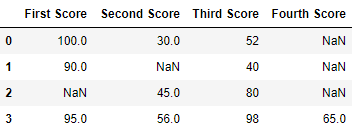Now we drop rows with at least one Nan value (Null value)

 `# importing pandas as pd``import` `pandas as pd`` ` `# importing numpy as np``import` `numpy as np`` ` `# dictionary of lists``dict` `=` `{``'First Score'``:[``100``, ``90``, np.nan, ``95``],``        ``'Second Score'``: [``30``, np.nan, ``45``, ``56``],``        ``'Third Score'``:[``52``, ``40``, ``80``, ``98``],``        ``'Fourth Score'``:[np.nan, np.nan, np.nan, ``65``]}`` ` `# creating a dataframe from dictionary``df ``=` `pd.DataFrame(``dict``)`` ` `# using dropna() function  ``df.dropna()`

Output: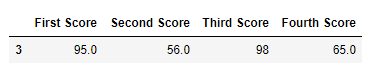Code #2: Dropping rows if all values in that row are missing.

 `# importing pandas as pd``import` `pandas as pd`` ` `# importing numpy as np``import` `numpy as np`` ` `# dictionary of lists``dict` `=` `{``'First Score'``:[``100``, np.nan, np.nan, ``95``],``        ``'Second Score'``: [``30``, np.nan, ``45``, ``56``],``        ``'Third Score'``:[``52``, np.nan, ``80``, ``98``],``        ``'Fourth Score'``:[np.nan, np.nan, np.nan, ``65``]}`` ` `# creating a dataframe from dictionary``df ``=` `pd.DataFrame(``dict``)``   ` `df`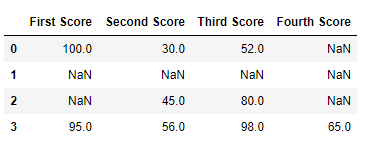Now we drop a rows whose all data is missing or contain null values(NaN)

 `# importing pandas as pd``import` `pandas as pd`` ` `# importing numpy as np``import` `numpy as np`` ` `# dictionary of lists``dict` `=` `{``'First Score'``:[``100``, np.nan, np.nan, ``95``],``        ``'Second Score'``: [``30``, np.nan, ``45``, ``56``],``        ``'Third Score'``:[``52``, np.nan, ``80``, ``98``],``        ``'Fourth Score'``:[np.nan, np.nan, np.nan, ``65``]}`` ` `df ``=` `pd.DataFrame(``dict``)`` ` `# using dropna() function    ``df.dropna(how ``=` `'all'``)`

Output: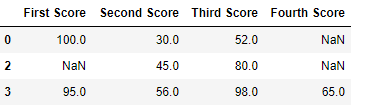Code #3: Dropping columns with at least 1 null value.

 `# importing pandas as pd``import` `pandas as pd``  ` `# importing numpy as np``import` `numpy as np``  ` `# dictionary of lists``dict` `=` `{``'First Score'``:[``100``, np.nan, np.nan, ``95``],``        ``'Second Score'``: [``30``, np.nan, ``45``, ``56``],``        ``'Third Score'``:[``52``, np.nan, ``80``, ``98``],``        ``'Fourth Score'``:[``60``, ``67``, ``68``, ``65``]}`` ` `# creating a dataframe from dictionary ``df ``=` `pd.DataFrame(``dict``)``    ` `df`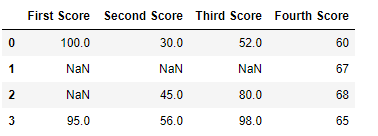Now we drop a columns which have at least 1 missing values

 `# importing pandas as pd``import` `pandas as pd``  ` `# importing numpy as np``import` `numpy as np``  ` `# dictionary of lists``dict` `=` `{``'First Score'``:[``100``, np.nan, np.nan, ``95``],``        ``'Second Score'``: [``30``, np.nan, ``45``, ``56``],``        ``'Third Score'``:[``52``, np.nan, ``80``, ``98``],``        ``'Fourth Score'``:[``60``, ``67``, ``68``, ``65``]}`` ` `# creating a dataframe from dictionary  ``df ``=` `pd.DataFrame(``dict``)`` ` `# using dropna() function     ``df.dropna(axis ``=` `1``)`

Output :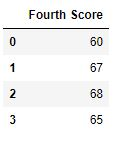Code #4: Dropping Rows with at least 1 null value in CSV file.

 `# importing pandas module ``import` `pandas as pd ``   ` `# making data frame from csv file ``data ``=` `pd.read_csv(``"employees.csv"``) ``   ` `# making new data frame with dropped NA values ``new_data ``=` `data.dropna(axis ``=` `0``, how ``=``'any'``) ``   ` `new_data`

Output: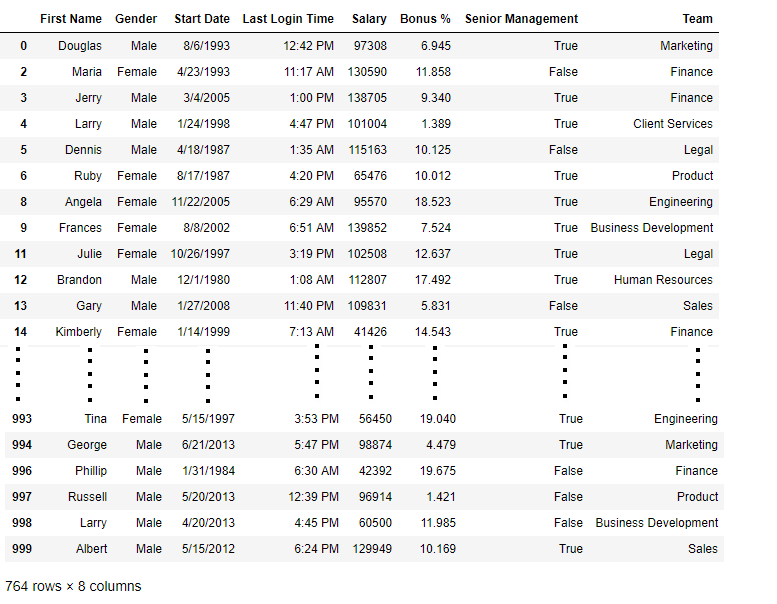Now we compare sizes of data frames so that we can come to know how many rows had at least 1 Null value

 `print``(``"Old data frame length:"``, ``len``(data))``print``(``"New data frame length:"``, ``len``(new_data)) ``print``(``"Number of rows with at least 1 NA value: "``,``      ``(``len``(data)``-``len``(new_data)))`

Output :

```Old data frame length: 1000
New data frame length: 764
Number of rows with at least 1 NA value:  236
```

Since the difference is 236, there were 236 rows which had at least 1 Null value in any column.

Attention geek! Strengthen your foundations with the Python Programming Foundation Course and learn the basics.

To begin with, your interview preparations Enhance your Data Structures concepts with the Python DS Course. And to begin with your Machine Learning Journey, join the Machine Learning – Basic Level Course

My Personal Notes arrow_drop_up